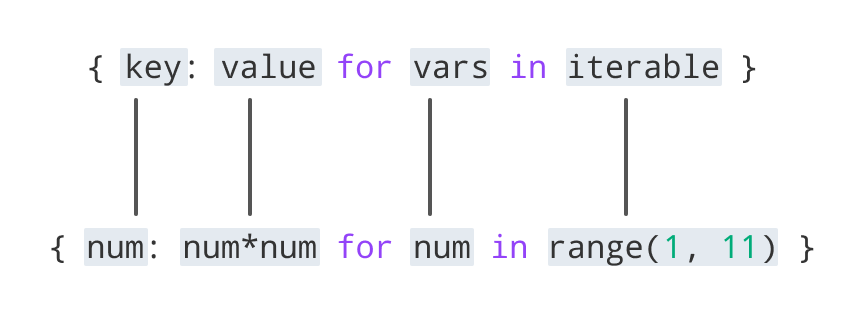# Python Dictionary Comprehension

Dictionaries are data types in Python which allows us to store data in key/value pair. For example:

``````my_dict = {1: 'apple', 2: 'ball'}
``````

## What is Dictionary Comprehension in Python?

Dictionary comprehension is an elegant and concise way to create dictionaries.

### Example 1: Dictionary Comprehension

Consider the following code:

``````square_dict = dict()
for num in range(1, 11):
square_dict[num] = num*num
print(square_dict)
``````

Now, let's create the dictionary in the above program using dictionary comprehension.

``````# dictionary comprehension example
square_dict = {num: num*num for num in range(1, 11)}
print(square_dict)
``````

The output of both programs will be the same.

`{1: 1, 2: 4, 3: 9, 4: 16, 5: 25, 6: 36, 7: 49, 8: 64, 9: 81, 10: 100}`

In both programs, we have created a dictionary `square_dict` with number-square key/value pair.

However, using dictionary comprehension allowed us to create a dictionary in a single line.

## Using Dictionary Comprehension

From the above example, we can see that dictionary comprehension should be written in a specific pattern.

The minimal syntax for dictionary comprehension is:

```dictionary = {key: value for vars in iterable}
```

Let's compare this syntax with dictionary comprehension from the above example.Now, let's see how we can use dictionary comprehension using data from another dictionary.

### Example 3: How to use Dictionary Comprehension

``````#item price in dollars
old_price = {'milk': 1.02, 'coffee': 2.5, 'bread': 2.5}

dollar_to_pound = 0.76
new_price = {item: value*dollar_to_pound for (item, value) in old_price.items()}
print(new_price)

``````

Output

```{'milk': 0.7752, 'coffee': 1.9, 'bread': 1.9}
```

Here, we can see that we retrieved the item prices in dollars and converted them to pounds. Using dictionary comprehension makes this task much simpler and shorter.

## Conditionals in Dictionary Comprehension

We can further customize dictionary comprehension by adding conditions to it. Let's look at an example.

### Example 4: If Conditional Dictionary Comprehension

``````original_dict = {'jack': 38, 'michael': 48, 'guido': 57, 'john': 33}

even_dict = {k: v for (k, v) in original_dict.items() if v % 2 == 0}
print(even_dict)
``````

Output

```{'jack': 38, 'michael': 48}
```

As we can see, only the items with even value have been added, because of the `if` clause in the dictionary comprehension.

### Example 5: Multiple if Conditional Dictionary Comprehension

``````original_dict = {'jack': 38, 'michael': 48, 'guido': 57, 'john': 33}

new_dict = {k: v for (k, v) in original_dict.items() if v % 2 != 0 if v < 40}
print(new_dict)
``````

Output

```{'john': 33}
```

In this case, only the items with an odd value of less than 40 have been added to the new dictionary.

It is because of the multiple `if` clauses in the dictionary comprehension. They are equivalent to `and` operation where both conditions have to be true.

### Example 6: if-else Conditional Dictionary Comprehension

``````original_dict = {'jack': 38, 'michael': 48, 'guido': 57, 'john': 33}

new_dict_1 = {k: ('old' if v > 40 else 'young')
for (k, v) in original_dict.items()}
print(new_dict_1)

``````

Output

```{'jack': 'young', 'michael': 'old', 'guido': 'old', 'john': 'young'}
```

In this case, a new dictionary is created via dictionary comprehension.

The items with a value of 40 or more have the value of 'old' while others have the value of 'young'.

## Nested Dictionary Comprehension

We can add dictionary comprehensions to dictionary comprehensions themselves to create nested dictionaries. Let's look at an example.

### Example 7: Nested Dictionary with Two Dictionary Comprehensions

``````dictionary = {
k1: {k2: k1 * k2 for k2 in range(1, 6)} for k1 in range(2, 5)
}
print(dictionary)
``````

Output

```{2: {1: 2, 2: 4, 3: 6, 4: 8, 5: 10},
3: {1: 3, 2: 6, 3: 9, 4: 12, 5: 15},
4: {1: 4, 2: 8, 3: 12, 4: 16, 5: 20}}
```

As you can see, we have constructed a multiplication table in a nested dictionary, for numbers from 2 to 4.

Whenever nested dictionary comprehension is used, Python first starts from the outer loop and then goes to the inner one.

So, the above code would be equivalent to:

``````dictionary = dict()
for k1 in range(11, 16):
dictionary[k1] = {k2: k1*k2 for k2 in range(1, 6)}
print(dictionary)
``````

It can further be unfolded:

``````dictionary = dict()
for k1 in range(11, 16):
dictionary[k1] = dict()
for k2 in range(1, 6):
dictionary[k1][k2] = k1*k2
print(dictionary)

``````

All these three programs give us the same output.

## Advantages of Using Dictionary Comprehension

As we can see, dictionary comprehension shortens the process of dictionary initialization by a lot. It makes the code more pythonic.

Using dictionary comprehension in our code can shorten the lines of code while keeping the logic intact.

## Warnings on Using Dictionary Comprehension

Even though dictionary comprehensions are great for writing elegant code that is easy to read, they are not always the right choice.

We must be careful while using them as :

• They can sometimes make the code run slower and consume more memory.
• They can also decrease the readability of the code.

We must not try to fit a difficult logic or a large number of dictionary comprehension inside them just for the sake of making the code single lined. In these cases, It is better to choose other alternatives like loops.